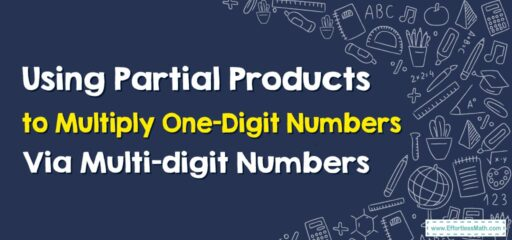# How to Use Partial Products to Multiply One-Digit Numbers By Multi-digit Numbers

Partial products are another great strategy for simplifying multiplication problems, especially when multiplying one-digit numbers by multi-digit numbers.## A Step-by-step Guide to Using Partial Products to Multiply One-Digit Numbers By Multi-digit Numbers

Here’s a step-by-step guide on how to use the partial products method to multiply one-digit numbers via multi-digit numbers:

Let’s use an example of 4 x 123.

### Step 1: Break down the multi-digit number

First, you need to break down the multi-digit number into its place values. In this case, 123 becomes $$100+20+3$$.

### Step 2: Multiply the single-digit number with each part of the multi-digit number

Now, you multiply the single-digit number (in this case, 4) with each part of the multi-digit number:

• $$4×100=400$$
• $$4×20=80$$
• $$4×3=12$$

### Step 3: Add the products

Finally, you add up all of these products to get the answer:
$$400+80+12=492$$
So, $$4×123=492$$

This method is called the Partial Products method because you are finding the product of parts of the multi-digit number (the “partial products”) and then adding them together. This method helps students understand the concept of multiplication and the importance of place value. It can be a great stepping stone before learning more traditional multiplication algorithms.

### What people say about "How to Use Partial Products to Multiply One-Digit Numbers By Multi-digit Numbers - Effortless Math: We Help Students Learn to LOVE Mathematics"?

No one replied yet.

X
30% OFF

Limited time only!

Save Over 30%

SAVE $5 It was$16.99 now it is \$11.99